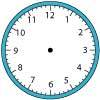You may also likeOn Time

On a clock the three hands - the second, minute and hour hands - are on the same axis. How often in a 24 hour day will the second hand be parallel to either of the two other hands?Right Time

At the time of writing the hour and minute hands of my clock are at right angles. How long will it be before they are at right angles again?Estimating Angles

How good are you at estimating angles?

Robo-turn

Age 11 to 14 Short Challenge Level:

45m

The total angle turned through after each of the first 4 moves is $10^{\circ}$, $30^{\circ}$, $60^{\circ}$ and $100^{\circ}$. So the robot does not face due East at the end of a move in its first complete revolution. The total angle it has turned through after each of the next 5 moves is $150^{\circ}$, $210^{\circ}$, $280^{\circ}$, $360^{\circ}$ and $450^{\circ}$, so at the end of the 9th move the robot does face due East. As the robot moves 5m in each move, the distance it travels is 45m.

Note that this solution assumes that the robot is not starting the process close to the North Pole!

This problem is taken from the UKMT Mathematical Challenges.
You can find more short problems, arranged by curriculum topic, in our short problems collection.